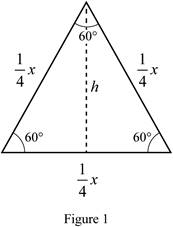# the volume of the monument.### Single Variable Calculus: Concepts...

4th Edition
James Stewart
Publisher: Cengage Learning
ISBN: 9781337687805### Single Variable Calculus: Concepts...

4th Edition
James Stewart
Publisher: Cengage Learning
ISBN: 9781337687805

#### Solutions

Chapter 6, Problem 21RE
To determine

## To find: the volume of the monument.

Expert Solution

The volume of the monument is 12533m3_.

### Explanation of Solution

Given information:

The height of the monument is 20m.

A horizontal cross section at a distance x meters from top is an equilateral triangle with side 14x meters.

Calculation:

Draw the equilateral triangle as shown in Figure 1.Refer to Figure 1.

Find the height of the equilateral triangle.

sin60°=h14xh=14x×sin60°=x4sin60°=38x

Find the area of triangle using the formula.

A(x)=12bh (1)

Here, b is breadth and h is height.

Substitute 14x for b, and 38x for h in Equation (1).

A(x)=12(14x)(38x)=364x2

Find the volume of monument using the formula:

V=abA(x)dx

Substitute 0 for a, 20 for b, and 364x2 for A(x)

V=020364x2dx=364020x2dx=364[x33]020=364

=364(8,0003)=12533

Therefore, volume of the monument is 12533m3_.

### Have a homework question?

Subscribe to bartleby learn! Ask subject matter experts 30 homework questions each month. Plus, you’ll have access to millions of step-by-step textbook answers!